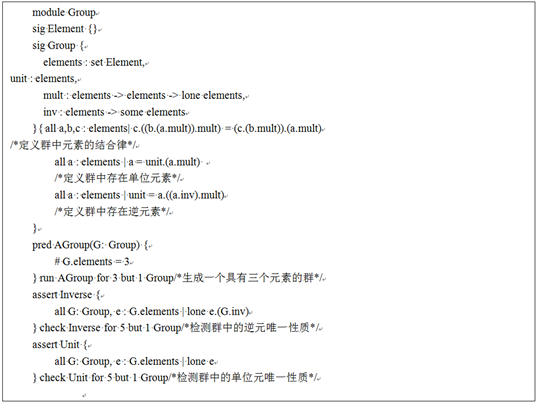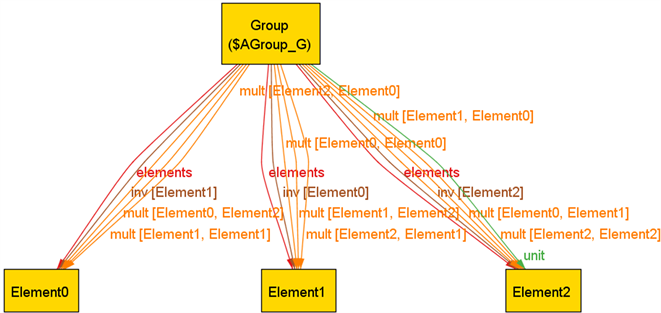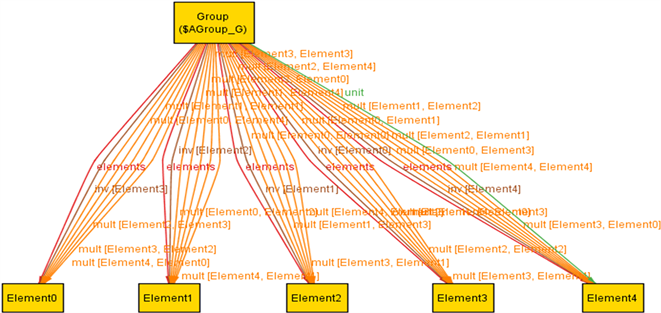# 基于Alloy的两个群定义的等价性验证Automated Verification of Equivalence of Two Group Definitions Based on Alloy

DOI: 10.12677/AAM.2017.69127, PDF, HTML, XML, 下载: 741  浏览: 1,018

Abstract: Group theory is an important part of algebraic system. This paper presents a new method to verify two Group definitions using Alloy. The two definitions of Group are formally described using Alloy, and the automatic verification of the two definitions of Group is realized by the Alloy analyzer. The experimental results show that the method is feasible and has high efficient.

1. 引言

FINDER、FALCON、LDPP、SATO等都属于早期的原型程序，到目前为止都已经没有了后续的工作。这些程序使用的语言表达能力有限。FINDER由于无法通过消除同构的方式来减小搜索空间，在计算机上运行的时间相对较长，不能高效地处理有关同构的群论定理。FALCON主要用于验证等式理论，只能应用于部分的群论定理。LDPP、SATO无法描述包含较长公式的定理   。总之，这些工具在验证群论定理的过程中都有一定的缺陷。

2. 预备知识

2.1. Alloy语言及Alloy分析器

Alloy语言是一种轻量级、基于关系的一阶逻辑语言，同时它也是说明性的建模语言。使用Alloy语言描述模型非常简洁，而且它可以表示所有数据类型的关系，包括集合、标量和元组，并通过列出属性和约束来描述一个系统的状态和行为，具有极强的描述能力   。其所建立的模型可以由Alloy分析器进行自动验证，并给出可视化结果。

Alloy分析器由MIT软件设计组开发，是基于模型检验理论的模型分析工具。它将模型转化为布尔表达式，然后使用可满足性理论(SAT Technology)对模型进行分析，进而进行模型检验  。Alloy分析器实际上是一个“模型查找”工具。只要给出一个Alloy语言的逻辑表达式，分析器将会在指定的范围内查找符合该逻辑表达式的模型，而这种逻辑表达式就是需要满足的属性。当这种属性不满足时，Alloy分析器就会产生对应的反例，用于辅助查找为什么会产生该属性不满足的现象。

2.2. 群的概念

(1) 结合律： $\left(ab\right)c=a\left(bc\right),\text{\hspace{0.17em}}a,b,c\in G$

(2) 存在单位元素：存在 $1\in G$ ，使对任意的 $a\in G$ ，恒有 $1a=a1=a$

(3) 存在逆元素：对任意的 $a\in G$ ，存在 ${a}^{-1}\in G$ ，使得 ${a}^{-1}a=a{a}^{-1}=1$

(1) 结合律： $\left(ab\right)c=a\left(bc\right),\text{\hspace{0.17em}}a,b,c\in G$

(2’) 存在左(右)单位元素：存在 $1\in G$ ，使对任意的 $a\in G$ ，恒有 $1a=a\left(a1=a\right)$

(3’) 存在左(右)逆元素：对任意的 $a\in G$ ，存在 ${a}^{-1}\in G$ ，使得 ${a}^{-1}a=1\left(a{a}^{-1}=1\right)$

3. 基于Alloy的群定义等价问题

3.1. 构建模型(1) 定义对象集合(Element及Group)，定义群中元素的三种关系(unit, mult, inv)；

(2) 描述了定义1中的三种约束关系；

(3) 谓词(AGroup)语句，生成一个具有三个元素的群(#在Alloy语言中代表个数)；

(4) 断言(Inverse)语句，在规定范围内检测上述群是否具有逆元唯一性质；

(5) 断言(Unit)语句，在规定范围内检测上述群是否具有单位元唯一性质。3.2. 实验结果

$Group$ 表示一个群， ${E}_{0}\text{\hspace{0.17em}},{E}_{1}\text{\hspace{0.17em}},{E}_{2}$ 分别表示群中的三个元素。观察上图中群的实例可知，其中 ${E}_{2}$ 表示该群中的单位元(unit)， ${E}_{0}\text{\hspace{0.17em}},{E}_{1}$ 互为逆元(inv)，且满足单位元唯一和逆元唯一的性质。

${E}_{0}\text{\hspace{0.17em}},{E}_{1}\text{\hspace{0.17em}},{E}_{2}\text{\hspace{0.17em}},{E}_{3}\text{\hspace{0.17em}},{E}_{4}$ 分别表示群中的五个元素。观察图中群的实例可知，其中 ${E}_{4}$ 表示该群中的单位元Figure 1. InstanceFigure 2. Instance

(unit)， ${E}_{0}\text{\hspace{0.17em}},{E}_{1}$ 互为逆元(inv)， ${E}_{2}\text{\hspace{0.17em}},{E}_{3}$ 互为逆元(inv)，且满足单位元唯一和逆元唯一的性质。

4. 结论

  张禾瑞. 近世代数基础[M]. 北京: 高等教育出版社, 2008.  Slaney, J. (1993) FINDER: Finite Domain Enu-merator. Version 3.0 Notes and Guide. Australian National University, Canberra.  Zhang, J. (1996) Constructing Finite Algebras with Falcon. Journal of Automated Reasoning, 16, 1-22. https://doi.org/10.1007/BF00244457  Zhang, H.T. and Stickel, M. (1994) Implementing the Davis-Putnam Algorithm by Tries. Technical Report, University of Iowa, Iowa City.  McCune, W. (1994) A Davis-Putnam Pro-gram and its Application to Finite First-Order Model Search: Quasigroup Existence Problems. Mathematics and Com-puter Science Division, Argonne National Laboratory, Argonne.  Zhang, J. and Zhang, H.T. (1995) SEM: A System for Enumerating Models. In: Department of Philosophy University of Wisconsin-Madison Mathematics and Computer Science, 298-303.  Torlak, E. and Jackson, D. (2007) Kodkod: A Relational Model Finder. Proceedings of the 13th International Conference on Tools and Algorithms for the Construction and Analysis of Systems, Braga, 632-647. https://doi.org/10.1007/978-3-540-71209-1_49  Jackson, D. (2006) Software Abstractions: Logic, Language, and Analysis. MIT Press, Cambridge, MA.  Jackson, D. (n.d.) Alloy: A Language & Tool for Relational Models. http://alloy.mit.edu/alloy  Jackson, D. (2000) Automating First-Order Relational Logic. ACM SIGSOFT Software Engineering Notes, 25, 130-139. https://doi.org/10.1145/357474.355063  Clarke, E.M. and Emerson, E.A. (1981) Design and Synthesis of Synchronization Skeletons Using Branching-time Temporal Logic. In: Logic of Pro-grams, Lecture Notes in Computer Science, Springer, 133: 52-71.  Torlak, E. and Dennis, G. (2006) Kodkod for Alloy Users. Massachusetts Institute of Technology Computer Science and Artificial Intelligence Laboratory, Cam-bridge.  聂灵沼, 丁石孙. 代数学引论[M]. 北京: 高等教育出版社, 2011: 23-25.  杨家海, 姜宁, 安长青, 李福亮. 基于形式化描述的交换机网络自动配置策略的设计与实现[J]. 清华大学学报(自然科学版), 2012(8): 1041-1048.